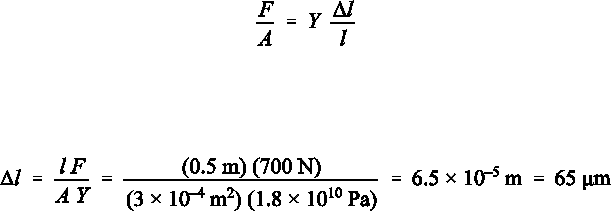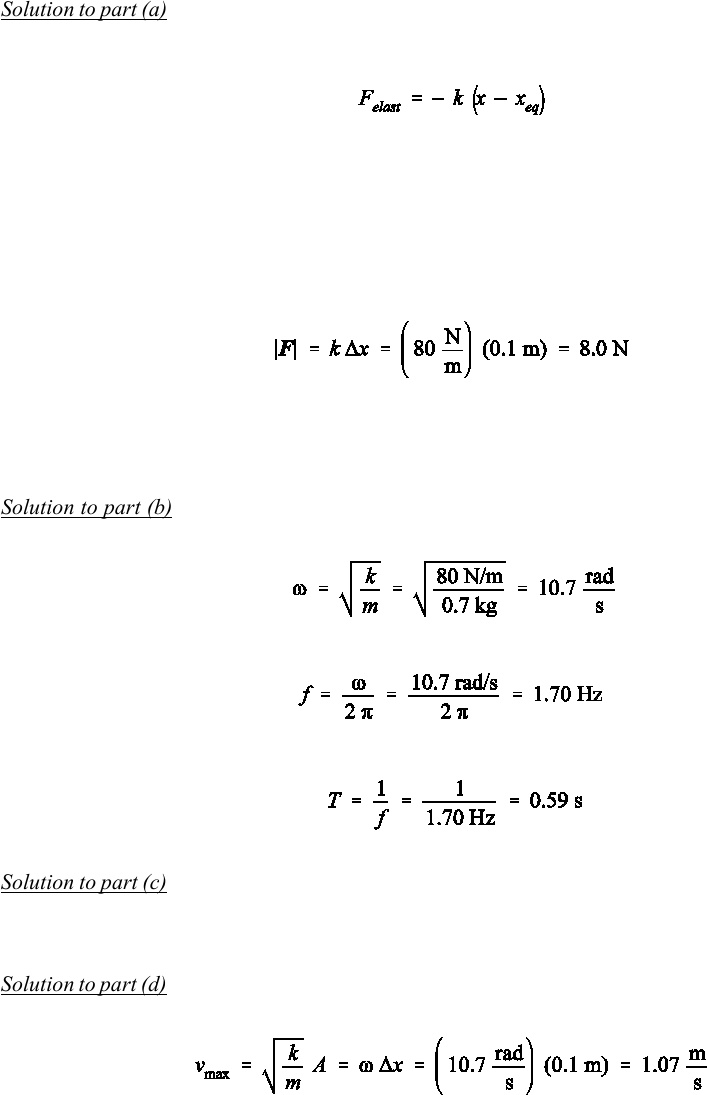# Physics 1028 Chapter Notes - Chapter 14: Angular Frequency, Net Force, Buoyancy

1 views6 pages
School
Western University
Department
Physics
Course
Physics 1028
ProfessorSelection of Even–Numbered Problem Solutions
Chapter 14
P–14.2
This is an application of Hooke’s law:
(1)
in which the force in the stress term F/A is given by the weight of the person. We are asked to calculate the
absolute change in length, l, which is part of the strain term l/l, in which l is the initial length of the bone.
Using the given value for Young's modulus Y, we find:
(2)
This is a tiny change in length!
P–14.4
The weight of the standard man is W = 690 N. It will create a pressure of 5.0 × 108 Pa when applied to a
cross–sectional area of A = W/p = 1.4 × 10–6 m². Since the wire has a circular cross–section the radius of the
wire will be derived from A = π r². Therefore the minimum diameter of the wire that supports a standard man
and still not exceed the given pressure is dmin = 1.3 mm.
Unlock document

This preview shows pages 1-2 of the document.
Unlock all 6 pages and 3 million more documents.P–14.6
Solution to part (a): We proceed with the assumption that the force acting on an object attached to the spring
in this problem is elastic, i.e., we use Hooke’s law. Hooke’s law is given for an object attached to a spring
as:
(3)
It is used in one of two ways: either both the equilibrium position and the actual position of the attached point
mass are given or are of interest. In this case x and xeq must be determined separately. Often, however, this
is not the case since we are only interested in the displacement from the equilibrium position, given as x =
xxeq. x is then a single parameter.
In the current problem, the equilibrium position is identified explicitly at the origin, meaning that
both ways to read Hooke’s law are identical with x = xm. Substituting the values given in Eq.  yields the
magnitude of the restoring force at x:
(4)
This force acts along the line between the equilibrium position and the actual position of the object, i.e., along
the x–axis in the current problem. The x–component of the force is Fx = – |F| = – 8.0 N, which is negative
because the force is a restoring force acting in the direction toward the equilibrium position of the spring.
Solution to part (b): The parameters sought in this part of the problem are all characteristic values for the
motion of an object attached to a specific spring. For the angular frequency ω we use its definition:
(5)
From Eq.  follows the frequency f:
(6)
and for the period:
(7)
Solution to part (c): The amplitude is obtained by finding the maximum displacement of the object from the
equilibrium position. Since it starts from rest, the position x(t = 0) represents the amplitude A of the vibration,
i.e., A = 10 cm.
Solution to part (d): The maximum speed is found at the time when the object passes through the equilibrium
position:
(8)
Unlock document

This preview shows pages 1-2 of the document.
Unlock all 6 pages and 3 million more documents.

## Document Summary

This is an application of hooke"s law: in which the force in the stress term f/a is given by the weight of the person. We are asked to calculate the absolute change in length, l, which is part of the strain term l/l, in which l is the initial length of the bone. Using the given value for young"s modulus y, we find: (1) (2) The weight of the standard man is w = 690 n. it will create a pressure of 5. 0 108 pa when applied to a cross sectional area of a = w/p = 1. 4 10 6 m . Since the wire has a circular cross section the radius of the wire will be derived from a = r . Therefore the minimum diameter of the wire that supports a standard man and still not exceed the given pressure is dmin = 1. 3 mm.